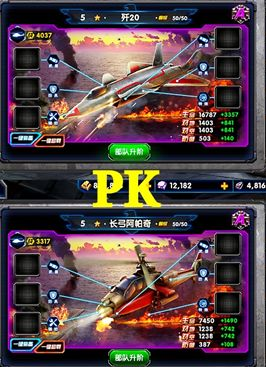## 新闻公告 当前位置：首页 >新闻公告 > 详细

### 【攻略】《最高警戒》威龙和长弓阿帕奇打首领数值对比（上28980×17=492660

492660×85%=418761

492660×150%=738990

492660×75%=369495

33240×12=398880

398880×150%=598320

398880×85%=339048

398880×120%=478656

75÷1.4=53次

28980×53=1535940

1535940×85%=1305549

1535940×150%=2303910

1535940×75%=1151955

100÷2=50次

33240×50=1662000

1662000×150%=2493000

1662000×85%=1412700

1662000×120%=1994400